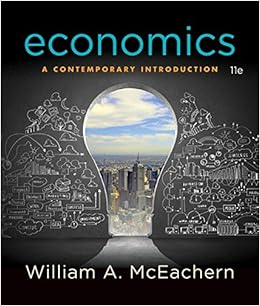# The model suggested that the price level would adjust

• Notes
• 16
• 100% (1) 1 out of 1 people found this document helpful

This preview shows page 8 - 10 out of 16 pages.

##### We have textbook solutions for you!
The document you are viewing contains questions related to this textbook.The document you are viewing contains questions related to this textbook.
Chapter 21 / Exercise 8
Economics: A Contemporary Introduction
McEachernExpert Verified
inflation model based on M2 growth. The model suggested that the price level would adjust to an equilibrium level, P * , that was determined mainly by the level of M2. See Jeffrey J. Hallman, Richard D. Porter, and David H. Small, “Is the Price Level Tied to the M2 Monetary Aggregate in the Long Run?” American Economic Review , September 1991, pp. 841–858. However, almost immediately after this article came out, M2 velocity began behaving very unpredictably, and the Federal Reserve deemphasized the use of M2 for policy purposes. G) Application: financial regulation, innovation, and the instability of money demand 1. Goldfeld (1973) found a stable money (M1) demand function 2. But late 1974 to early 1976, M1 demand fell relative to that predicted by the model 3. And in the early 1980s, M1 demand rose relative to that predicted by the model 4. Why did money demand shift erratically? Increased innovation and changes in the financial system (see text Figure 7.2) a. New assets were invented in the 1970s, liquid assets that paid interest People switched wealth from M1 to these assets, reducing M1 demand (1) MMMFs (2) Overnight repurchase agreements b. New assets in the 1980s, interest-bearing checking accounts; their use brought wealth into M1, raising money demand 5. Developments in the 1990s a. Sweep programs reduce demand for reserves and M1 b. M2 erratic because of increased use of mutual funds Policy Application The Federal Reserve’s job of conducting monetary policy is made more complicated by sweep programs. For an introduction to these problems, see “Sweep Accounts Lower Reserve Balances, Complicate Fed Funds Targeting” in the Federal Reserve Bank of Atlanta Financial Update , July–September 1999.
##### We have textbook solutions for you!
The document you are viewing contains questions related to this textbook.The document you are viewing contains questions related to this textbook.
Chapter 21 / Exercise 8
Economics: A Contemporary Introduction
McEachernExpert Verified
Chapter 7 The Asset Market, Money, and Prices 129 IV. Asset Market Equilibrium (Sec. 7.4) A) Asset market equilibrium—an aggregation assumption 1. Assume that all assets can be grouped into two categories, money and nonmonetary assets a. Money includes currency and checking accounts (1) Pays interest rate i m (2) Supply is fixed at M b. Nonmonetary assets include stocks, bonds, land, etc. (1) Pays interest rate i = r + π e (2) Supply is fixed at NM 2. Asset market equilibrium occurs when quantity of money supplied equals quantity of money demanded a. m d + nm d = total nominal wealth of an individual b. M d + NM d = aggregate nominal wealth (from adding up individual wealth) (7.6) c. M + NM = aggregate nominal wealth (supply of assets) (7.7) d. Subtracting (7.7) from (7.6) gives ( M d M ) + ( NM d NM ) = 0 (7.8) e. So the excess demand for money ( M d M ) plus the excess demand for nonmonetary assets ( NM d NM ) equals 0. f. So if money supply equals money demand, nonmonetary asset supply must equal nonmonetary asset demand; then the entire asset market is in equilibrium B) The asset market equilibrium condition 1. M / P = L ( Y , r + π e ) (7.9) real money supply = real money demand a. M is determined by the central bank b. π e is fixed (for now) c. The labor market determines the level of employment; using employment in the production function determines Y d. Given Y , the goods market equilibrium condition determines r Numerical Problem 2 is an exercise in calculating the equilibrium interest rate.
•••